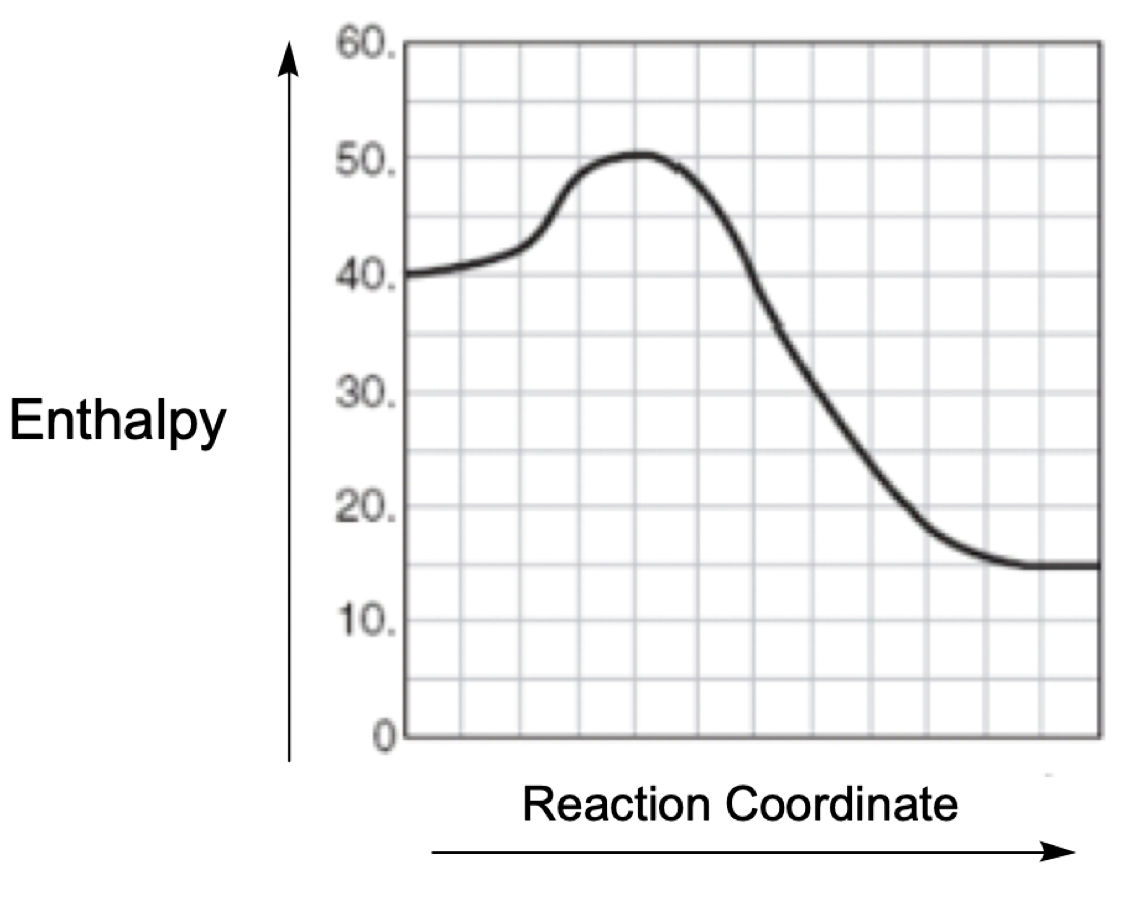Clutch Prep is now a part of Pearson
Ch.13 - Chemical KineticsWorksheetSee all chapters

# Catalyst

See all sections
Sections
Intro to Chemical Kinetics
Energy Diagrams
Catalyst
Factors Influencing Rates
Average Rate of Reaction
Stoichiometric Rate Calculations
Instantaneous Rate
Collision Theory
Arrhenius Equation
Rate Law
Reaction Mechanism
Integrated Rate Law
Half-Life

Catalyst is a substance that increases the rate of reaction by lowering the Activation Energy (Ea) of a reaction.

###### Catalyzed vs Uncatalyzed Reactions

Concept #1: Catalyzed vs Uncatalyzed Reactions

Example #1: A certain reaction has an enthalpy value of –20 kJ and an activation energy of 40 kJ. A catalyst is found that lowers the activation energy of the reaction by 10 kJ. What is the total difference in energy between the products and the transition state?

Practice: Which of the following statements is true regarding the energy diagram provided?

i. The reaction is endothermic.

ii. Activation energy would be less than + 10 kJ after a catalyst is added.

iii. The reaction absorbs energy.

iv. Activation energy would be greater than + 10 kJ after a catalyst is added.a) I only            b) II only      c) I and III         d) II, III and IV